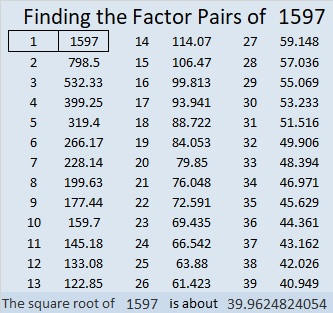# 1597 and Level 3

Contents

### Today’s Puzzle:

You can solve this level 3 puzzle! Each number from 1 to 10 must appear in both the first column and the top row.

What is the greatest common factor of 24 and 56? Write that number above the column in which those clues appear. Write the corresponding factors in the first column. Next, starting with 72, write the factors of each clue going down the puzzle row by row until you have found all the factors.### Factors of 1597:

• 1597 is a prime number.
• Prime factorization: 1597 is prime.
• 1597 has no exponents greater than 1 in its prime factorization, so √1597 cannot be simplified.
• The exponent in the prime factorization is 1. Adding one to that exponent we get (1 + 1) = 2. Therefore 1597 has exactly 2 factors.
• The factors of 1597 are outlined with their factor pair partners in the graphic below.How do we know that 1597 is a prime number? If 1597 were not a prime number, then it would be divisible by at least one prime number less than or equal to √1597. Since 1597 cannot be divided evenly by 2, 3, 5, 7, 11, 13, 17, 19, 23, 29, 31, or 37, we know that 1597 is a prime number.

### More about the Number 1597:

1597 is the 17th Fibonacci number. It is also the only 4-digit Fibonacci prime.

1597 is the sum of two squares:
34² + 21² = 1597.

1597 is the hypotenuse of a Pythagorean triple:
715-1428-1597, calculated from 34² – 21², 2(34)(21), 34² + 21².

Here’s another way we know that 1597 is a prime number: Since its last two digits divided by 4 leave a remainder of 1, and 34² + 21² = 1597 with 34 and 21 having no common prime factors, 1597 will be prime unless it is divisible by a prime number Pythagorean triple hypotenuse less than or equal to √1597. Since 1597 is not divisible by 5, 13, 17, 29, or 37, we know that 1597 is a prime number.

This site uses Akismet to reduce spam. Learn how your comment data is processed.